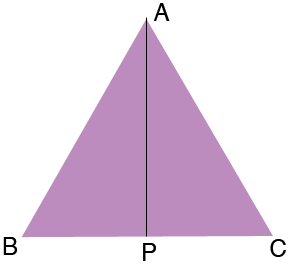SEARCH HOMEMath Central Quandaries & QueriesQuestion from ginny, a student: If a equilateral triangle has side lengths of 41.33m how would i calculate the area? if i don't know the height of the triangle? thank you.Ginny,

In my diagram P is the midpoint of BC.Triangles APC and ABP are congruent. Can you see why? This means that angle APC is a right angle and hence you can use the Theorem of Pythagoras to find |AP| which is the height of the triangle.

PennyMath Central is supported by the University of Regina and The Pacific Institute for the Mathematical Sciences.